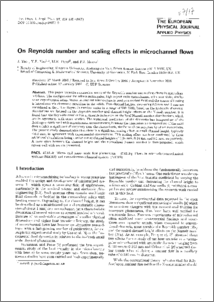# On Reynolds number and scaling effects in microchannel flows

Yao, Jun, Yao, Yufeng, Patel, Mayur and Mason, Peter J. (2007) On Reynolds number and scaling effects in microchannel flows. The European Physical Journal Applied Physics, 37 (2). pp. 229-235. ISSN 1286-0042 (doi:https://doi.org/10.1051/epjap:2007010)Preview
PDF
07_17.pdf

## Abstract

This paper presents a numerical study of the Reynolds number and scaling effects in microchannel flows. The configuration includes a rectangular, high-aspect ratio microchannel with heat sinks, similar to an experimental setup. Water at ambient temperature is used as a coolant fluid and the source of heating is introduced via electronic cartridges in the solids. Two channel heights, measuring 0.3 mm and 1 mm are considered at first. The Reynolds number varies in a range of 500-2200, based on the hydraulic diameter. Simulations are focused on the Reynolds number and channel height effects on the Nusselt number. It is found that the Reynolds number has noticeable influences on the local Nusselt number distributions, which are in agreement with other studies. The numerical predictions of the dimensionless temperature of the fluid agree fairly well with experimental measurements; however the dimensionless temperature of the solid does exhibit a significant discrepancy near the channel exit, similar to those reported by other researchers. The present study demonstrates that there is a significant scaling effect at small channel height, typically 0.3 mm, in agreement with experimental observations. This scaling effect has been confirmed by three additional simulations being carried out at channel heights of 0.24 mm, 0.14 mm and 0.1 mm, respectively. A correlation between the channel height and the normalized Nusselt number is thus proposed, which agrees well with results presented.

Item Type: Article Reynolds number, heat sinks, heat exchangers, Nusselt number, heat transfer, digital simulation, modelling, rectangular pipe, scaling laws, microfluidics, pipe flow Q Science > QA Mathematics > QA75 Electronic computers. Computer science School of Computing & Mathematical Sciences > Centre for Numerical Modelling & Process AnalysisSchool of Computing & Mathematical SciencesSchool of Computing & Mathematical Sciences > Centre for Numerical Modelling & Process Analysis > Computational Mechanics & Reliability GroupSchool of Computing & Mathematical Sciences > Department of Mathematical SciencesSchool of Computing & Mathematical Sciences > Centre for Numerical Modelling & Process Analysis > Fire Safety Engineering GroupSchool of Computing & Mathematical Sciences > Centre for Numerical Modelling & Process Analysis > Computational Science & Engineering Group 14 Oct 2016 09:03 http://gala.gre.ac.uk/id/eprint/1077View Item